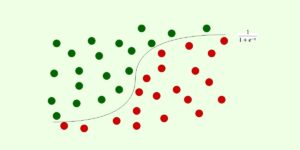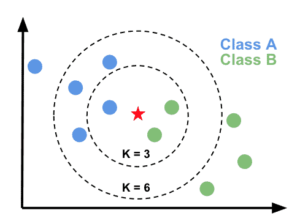Logistic Regression vs. K Nearest Neighbors in Machine LearningMl wizard

In machine learning, using an appropriate algorithm according to the behavior of the data and its pattern is an essential thing to achieve higher accuracies and accurate performing models. Many machine learning algorithms can be used for almost all types of problem statements in machine learning. The best-performing among them can be selected based on performance and accuracy.

There is some algorithm that is famous and known for their best performance and hence used the most, but still, they have their PROs and CONs. Knowing this information about the algorithms may help one to select an appropriate algorithm according to the data and problem statement and can also help to answer interview questions as well.

In this article, we will discuss logistic regression, KNN and KMeans algorithm, their PROs and CONs with the understanding of every advantage and disadvantage.

# Table of Content

1. Logistic Regression
2. KNN
3. Which to Use?
4. Key Takeaways
5. Conclusion

# 1. Logistic RegressionImage Source

Logistic regression in machine learning is a classification algorithm that is mainly used for binary and multivariate classification problems. This algorithm works on the principle of maximum likelihood estimation, where the maximization of the likelihood function is performed, and the best-fit line is obtained according to the distribution of the data on the plot. In the deep learning approach, the algorithm is also known as the Perceptron trick algorithm.

## PROs:

### Overfitting

The algorithm is significantly less prone to overfitting, and it is observed that using logistic regression with any type of dataset (Except high dimensional) will not lead to overfitting and handle such types of problems easily.

### Easy and Efficient

The algorithm is very easy to understand, and the training of the same is very efficient, where the time complexity associated with the logistic regression is very less compared to other machine learning algorithms.

### Performance

The algorithm performs very well when the data is linearly separate=able, meaning that the logistic regression algorithm performs very well when the data is linear and can be separated by just a linear line.

## CONs:

### High-Dimensional Data

Although the logistic regression algorithm is known for being less prone to overfitting, in the case of very high dimensional data where the features of the dataset are very high and the dataset is large and complex, the algorithm overfits the data and performs poorly on the testing data.

### Performance

Similar to the assumption of linear regression, this algorithm also should not be used when the number of observations is less than the number of features in the dataset. The algorithm performs very poorly in such types of cases.

### Non-Linear Datasets

The logistic regression algorithm can be used when the dataset is linear and can be separated by a linear line, but the algorithm does not perform that well when the distribution of the dataset is complex and the dataset is not linear.

# 2. K Nearest NeighboursImage Source

The K nearest Neighbors in machine learning is a distance-based working algorithm, which calculates the distance between the data points and tries to work according to the distribution of the dataset. This algorithm is used for regression and classification problems and almost always performs best in both cases.

The KNN algorithm is known as the lazy learning algorithm. This is because the algorithm does not train during the training stage. The KNN algorithm just stores the data when the data is fed to the algorithm in the training stage, and at the time of prediction, the algorithm tries to apply all the mechanisms it uses, and the prediction is made.

## PROs:

### Time Complexity

The KNN algorithm is a lazy learning algorithm that does not do anything in the training phase and makes all the calculations in the testing phase. Hence the training time in KNN is also significantly less. That is the main reason behind the algorithm’s slower predictions and faster training.

### Uses cases

The KNN algorithm can be used for both regression and classification problems and can be used very easily by implementing the same using the SKLearn library.

### No Training

The algorithm does not train during the training phase and hence the training is very fast in this algorithm, hence it is said that there is no training in the KNN algorithm.

## CONs:

### Slower Prediction

As discussed above, the algorithm is a lazy learning algorithm that makes all the calculations in the testing phase. Hence, the predictions in the KNN algorithm are very slow and take time.

### Correct K Value

Choosing the correct K value in the KNN algorithm is also an essential thing. If the selected K value is not optimal, then the algorithm will not perform well and need hyperparameter tuning.

### Feature Scaling

The algorithm works on the principle of calculating the distances between the data points, now here, in this case, the scale of the features that are present in the dataset can be different and very far away. In this case, the distances will be very high and the algorithm’s performance will be biased. So it is necessary to scale the features using feature scaling before applying the KNN algorithm to any dataset.

### Outliers

As we know that outliers are data points that are very different from all the other data points present in the data. The critical values of the outliers can affect the performance of the KNN algorithm and they need to be handled before using the KNN algorithm.

# Which to Use?

Both of the algorithms are well known for their performance and accuracy, but they should be used in some cases where it performs better compared to other algorithms, and the decision should be taken according to the behavior of the data and data patterns. For example, logistic regression can be used for only classification, and KNN can be used for both. The KNN can be used when there is a need for faster training. In the case of outliers, the outliers should be treated before training in KNN, or we Can use logistic regression if it’s a classification problem, etc.

# Key Takeaways

1. Logistic Regression is a classification algorithm that works on the principle of maximum likelihood estimation.
2. The Logistic algorithm performs well on linear data and is very easy to implement.
3. The KNN algorithm is a lazy learning algorithm that makes predictions during the testing phase and just stores the data during the training phase.
4. The KNN algorithm is affected by the outlier most, and hence the outliers should be treated before training on the data.

# Conclusion

In this article, we discussed the logistic regression and KNN algorithm with their intuition, PROs, and CONs associated with them. This will help one select the appropriate algorithm and know the various advantages and disadvantages of the algorithms.